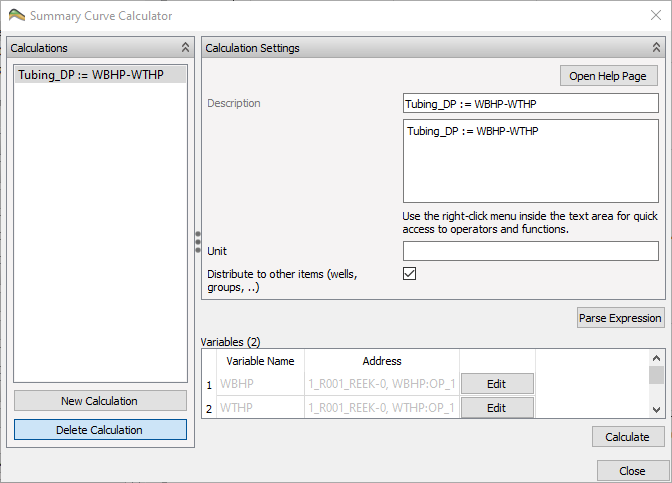# Calculator ExpressionsTwo similar calculators use expressions, summary curve calculator and grid cell calculator. These two variants share the text parsing for calculator expressions.

Grid Property Calculator

Summary Curve Calculator

ResInsight supports a subset of the features supported in the exprtk parsing library. Scripting features like for/while loops are not supported. The full documentation for the expression parser is available at C++ Mathematical Expression Toolkit Library

## Operators and Functions

Possible operations and functions are found by right-clicking in the expression window.

### Comment lines

Create a comment line by prefixing with // or ##

// Valid comment line 2
## Valid comment line 1


### Assignment Operators

OPERATOR DEFINITION
:= Assignment
## Example
MY_VARIABLE := b + c


### Basic Operators

OPERATOR DEFINITION
- Subtraction
* Multiplication
/ Division
% Modulus
^ Power
## Example
a := (b * c) + d / 10.5


### Conditionals

if-then-else statements can be used to assign values based on the individual values in a vector. The following case will assign 0.01 if the TRANX is below 0.01, else copy the original TRANX value.

## Example
NEW_TRANX := if((TRANX < 0.01), 0.01, TRANX)


### Scalar Functions

FUNCTION DEFINITION
avg Average
max Maximum
min Minimum
sum Sum
## Example: Use the accumulated sum for all PORV values to compute the normalized PORV
NORMALIZED_PORV := x/sum(PORV)


### Trigonometry Functions

FUNCTION DEFINITION
acosh Inverse hyperbolic cosine (in radians)
asinh Inverse hyperbolic sine (in radians)
atanh Inverse hyperbolic tangent (in radians)
cos Cosine
cosh Hyperbolic cosine
cot Cotangent
csc Cosecant
sec Secant
sin Sine
sinc Sine cardinal
sinh Hyperbolic sine
tan Tangent
tanh Hyperbolic tangent

### Vector Functions

FUNCTION DEFINITION
abs Absolute value
ceil Rounding up
floor Rounding down
frac Fractional portion of input
log Natural logarithm
log10 Base 10 logarithm
pow Power
round Round x to the nearest integer
sgn Sign of x, -1 where x < 0, +1 where x > 0, else zero
sqrt Square root
trunc Integer portion of input